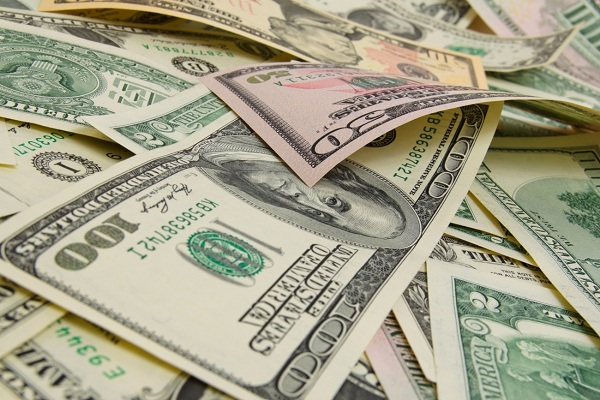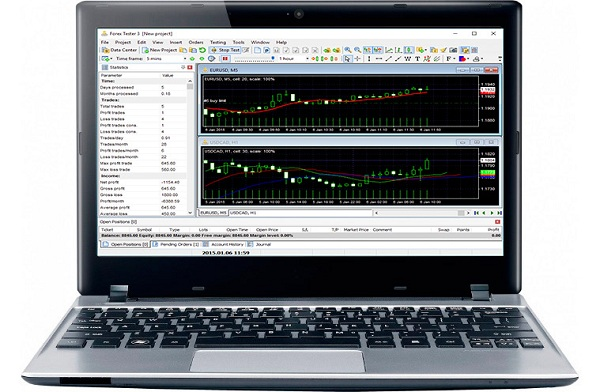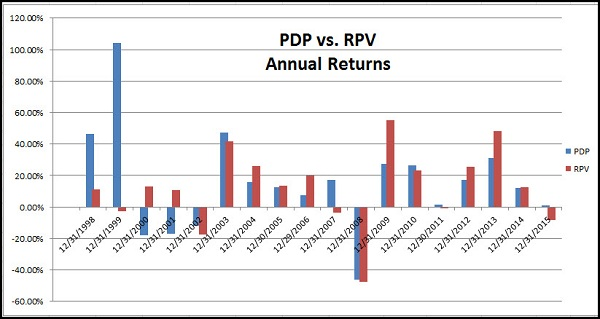Essential Property Surveillance Tools for Business2 Simple Moving TipsThings to Do on Bald Head IslandTypes of AerationHow to Choose the Perfect Office Chair
Home » Investment » Testing Profitable Investments

# Testing Profitable Investments

This is the second part of the technique to know if our investments are profitable. In the previous article detailing how to use Excel to analyze historical results. In this one, we will check Montecarlo’s distribution of the results, which will allow us to know the probability that the results will be repeated in the future.

As before, we will use historical data from an earlier version of Slowinver as the basis for the study.We already verified that the system obtained an annual profitability of the order of 29% and a maximum streak of losses of 15%, a very good result.

The million dollar question is: Will these results be repeated in the future?

One way to find out is to apply a Monte Carlo test.

The idea of Montecarlo is very simple, let’s see it with an example.

Suppose we use an investment method that obtains these results in 21 days:

Example montecarlo slowinver

In the data on the left, we can see that up to day 17 (in yellow) we get a cumulative 5.19%. And after the 21 operations, 6.6%.

In the data on the right, we randomly randomized the days. Since the results are the same, the final result is still 6.6%. However, on day 17 loses 3.4%, e.g. 8% worse than the original day 17.

The reason is that the sequence of operations until day 17 is worse in the 2nd case, and the two results tables are, in principle, just as likely.The idea therefore of the proof of Montecarlo is to make these disarrangements, not once, but hundreds or thousands of times. Each one of them will give us a different result curve in the intermediate terms; The typical Monte Carlo chart looks like this:

• All benefit curves are disordered outcomes, that is, they have all the same basic statistics in terms of mathematical hope, probability of success, volatility, etc., and are all equiprobables. Although only one of them is the original data curve.
• The dispersion of the different lines gives us an idea of the possible future deviation of our results from the past results. Therefore, the smaller the deviation of the Montecarlo analysis, the better.

I have applied Montecarlo to the historical results of 11 years of the system, messing it up 1000 times. Thus, I get 1000 result curves, and each represents the 11 years.

However, it has never seemed very practical to me to represent a graph of curves like the previous example. Instead of displaying graphs 1000, we will study their frequencies results in a histogram, just as we did in the previous article

. Thus we will see how many graphs of 10 years return a profitability of -5%, how many of 0%, how many of 5%, how many of 10%, etc.

Therefore, unlike the previous article, this histogram is not a daily result, but results from 1000 Monte Carlo tests.In The horizontal x – axis shows the annual compound return (CAR) as by 1. That is each value, the average annual result of 11 years of operations .

The lowest value is 0.90 e.g. -10%. The value 1 means to stay abreast. The highest value is around 1.95, which for 1 means 95% annual return average.

Between the two extremes there are 998 yields averaged annually.

The most common profitability (fashion) is around 32% . Although the average arithmetic of all Monte Carlo simulations is a return of 28%, very similar to the result of the original curve.

Summary-slowinver-CARIn addition to the average, we can draw other conclusions from this Monte Carlo, analyzing the pink line of cumulative probability. We can highlight these 3:

The system is profitable in a year with a 95% : the number Montecarlos ranging between 0% and 100% return.

The system obtains yields acceptable or even better (above 14%), 77.5% of the years, more than 3 out of 4 years.

The system obtains yields extraordinary (over 40%) 24.7% of the year (1 in 4)

The study, therefore, of the histogram allows us to have realistic expectations.

But although returns are interesting, it is also important to look at the maximum Drawdowns ( draw down ). The chart of loss ratios of the 1000 simulations of Montecarlo is:

Montecarlo-slowinver-DD

The smallest “worst losing streak” (Max DD) is 0.04 (4% losing streak), and the maximum, 22%.

Recall that each value represents the worst losing streak in almost 11 years.

Again, we obtain conclusions regarding the expected DDs:

The average maximum losses spurts is 9.1% , a very tolerable figure.

We can infer the DD of the future. With a reliability of 95%, they will be between 3% and 17%.

Overcoming the 20% of DD occurs 1.5% of the years, one year out of 66.

So in any investment there is risk, but the Montecarlo test gives us a pretty rough idea of what that risk is. Ultimately any investment is a matter of numbers, which we must analyze.

There are other studies to check the reliability of systems, but this spreadsheet will allow us to know in depth an investment method without devoting excessive time or very complex programs.

Since the sheet is based on daily results, we can enter results of any asset or investment method. For example, many funds allow you to lower your daily results from your web pages. By copying and pasting that data into the sheet, we will have a more accurate knowledge of its reliability.

Example Excel

I have done it with some well-known backgrounds, with certainly surprising results.Hi, I am Andrew Chowdhury; I am an entrepreneur, father, mentor and adventurer passionate about life. At this moment, I am working with depression and anxiety; here is my blogs how to recover from anxiety and how to fight with anxiety. I hope everyone will like my blogs.